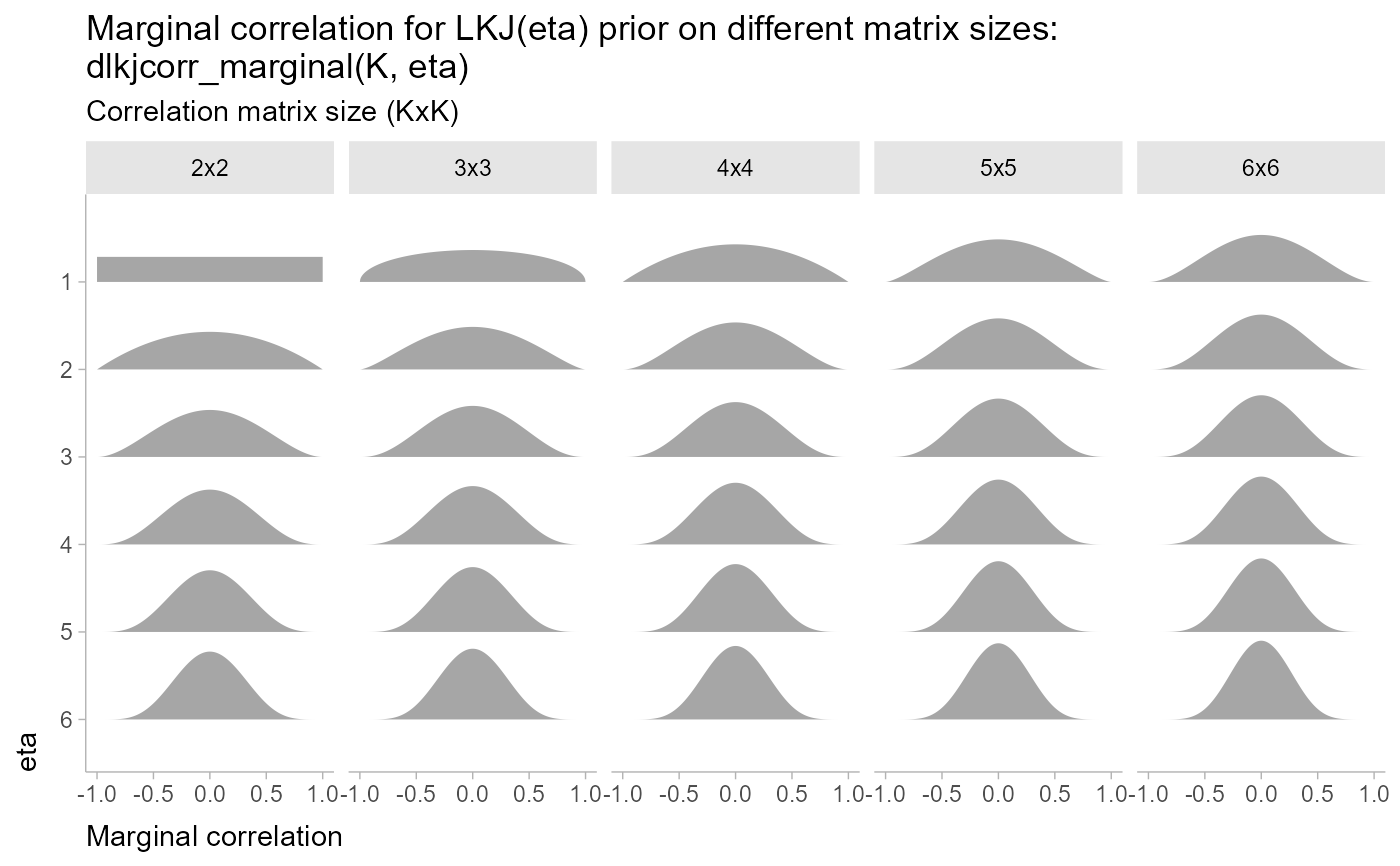Marginal distribution for the correlation in a single cell from a correlation matrix distributed according to an LKJ distribution.

dlkjcorr_marginal(x, K, eta, log = FALSE)

plkjcorr_marginal(q, K, eta, lower.tail = TRUE, log.p = FALSE)

qlkjcorr_marginal(p, K, eta, lower.tail = TRUE, log.p = FALSE)

rlkjcorr_marginal(n, K, eta)

## Arguments

x, q

vector of quantiles.

K

Dimension of the correlation matrix. Must be greater than or equal to 2.

eta

Parameter controlling the shape of the distribution

log, log.p

logical; if TRUE, probabilities p are given as log(p).

lower.tail

logical; if TRUE (default), probabilities are $$P[X \le x]$$ otherwise, $$P[X > x]$$.

p

vector of probabilities.

n

number of observations. If length(n) > 1, the length is taken to be the number required.

## Value

• dlkjcorr_marginal gives the density

• plkjcorr_marginal gives the cumulative distribution function (CDF)

• qlkjcorr_marginal gives the quantile function (inverse CDF)

• rlkjcorr_marginal generates random draws.

The length of the result is determined by n for rlkjcorr_marginal, and is the maximum of the lengths of the numerical arguments for the other functions.

The numerical arguments other than n are recycled to the length of the result. Only the first elements of the logical arguments are used.

## Details

The LKJ distribution is a distribution over correlation matrices with a single parameter, $$\eta$$. For a given $$\eta$$ and a $$K \times K$$ correlation matrix $$R$$:

$$R \sim \textrm{LKJ}(\eta)$$

Each off-diagonal entry of $$R$$, $$r_{ij}: i \ne j$$, has the following marginal distribution (Lewandowski, Kurowicka, and Joe 2009):

$$\frac{r_{ij} + 1}{2} \sim \textrm{Beta}\left(\eta - 1 + \frac{K}{2}, \eta - 1 + \frac{K}{2}\right)$$

In other words, $$r_{ij}$$ is marginally distributed according to the above Beta distribution scaled into $$(-1,1)$$.

parse_dist() and marginalize_lkjcorr() for parsing specs that use the LKJ correlation distribution and the stat_slabinterval() family of stats for visualizing them.

## Examples


library(dplyr)
library(ggplot2)
library(forcats)

theme_set(theme_ggdist())

expand.grid(
eta = 1:6,
K = 2:6
) %>%
ggplot(aes(y = fct_rev(ordered(eta)), dist = "lkjcorr_marginal", arg1 = K, arg2 = eta)) +
stat_slab() +
facet_grid(~ paste0(K, "x", K)) +
labs(
title = paste0(
"Marginal correlation for LKJ(eta) prior on different matrix sizes:\n",
"dlkjcorr_marginal(K, eta)"
),
subtitle = "Correlation matrix size (KxK)",
y = "eta",
x = "Marginal correlation"
) +
theme(axis.title = element_text(hjust = 0))